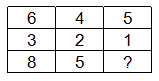Question 189

# Select the missing number from the given responses.Solution

Let number in first row be 'x' , in second row be 'y' and in third row be 'z'

The pattern followed is : $$x + y - 1 = z$$

=> The missing number = 5 + 1 - 1 = 5

Ans - (B)

• Free SSC Study Material - 18000 Questions
• 230+ SSC previous papers with solutions PDF